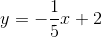Scan QR code or get instant email to install app

Question:

# Which of the following correctly describes the relationship between the two linear equations: 3y - 2x + 2 = 13x - 19 and x + 5y = 10

A perpendicular
explanation

1212Rewrite each equation in slope-intercept form and compare their slopes: 3y - 2x + 2 = 13x - 19, adding 2x - 2 to both sides 3y = 15x - 21, dividing by 3 y = 5x - 7 And: x + 5y = 10, subtracting x from both sides 5y = -x + 10, dividing by 5The slopes are negative reciprocals of each other; the lines are perpendicular to each other.